PeakEQ

# PeakEQ

### Overview

PeakEQ is an implementation of a single peaking equalizer filter, with sweepable frequency, bandwidth, and gain. When used in series, the PeakEQ filter can be used to construct parametric and graphic equalizers commonly found in DAWs.

### Algorithm Details

The filter design based on the 2nd-order equalization filter design by Reglia and Mitra, from the paper "Tunable Digital Frequency Response Equalization Filters", IEEE Trans. on Ac., Sp. and Sig Proc., 35 (1), 1987

This implementation takes the original filter design and converts it to a direct-form 2 filter configuration, which has better numerical stability.

### Conversion to Direct Form 2

We begin with the transfer function, described in the 1987 paper: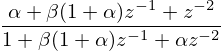This has the following difference equation: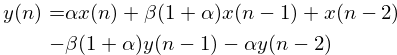The Direct Form II Structure. can be represented in this generalized difference equation: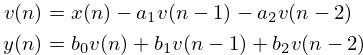Converting to direct form II is a matter of putting coefficients in their correct places. Placing in the coefficients from the Reglia and Mitra design yields the following difference equation: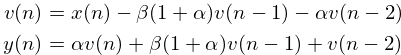Which can be translated to C code.

### Tangled Files

Tangles to files peakeq.c and peakeq.h.

<<peakeq.c>>=
#include <math.h>
#define SK_PEAKEQ_PRIV
#include "peakeq.h"

#ifndef M_PI
#define M_PI 3.14159265358979323846
#endif

<<funcs>>

When SK_PEAKEQ_PRIV is defined, it exposes the main struct.

<<peakeq.h>>=
#ifndef SK_PEAKEQ_H
#define SK_PEAKEQ_H

#ifndef SKFLT
#define SKFLT float
#endif

<<typedefs>>
<<funcdefs>>

#ifdef SK_PEAKEQ_PRIV
<<structs>>
#endif
#endif

### Struct

Everything is stored in a struct called sk_peakeq.

<<typedefs>>=
typedef struct sk_peakeq sk_peakeq;

<<structs>>=
struct sk_peakeq {
<<sk_peakeq>>
};

### Init

PeakEQ is initialized with sk_peakeq_init. The sampling rate is required here.

<<funcdefs>>=
void sk_peakeq_init(sk_peakeq *eq, int sr);

<<funcs>>=
void sk_peakeq_init(sk_peakeq *eq, int sr)
{
<<init>>
}

### Filter State Memory and Constants

The direct-form 2 form can be thought of as a 2-pole filter, followed by a 2-zero filter.

The memory for the 2-pole filter is stored in a variable called v.

<<sk_peakeq>>=
SKFLT v;

<<init>>=
eq->v = 0;
eq->v = 0;

The alpha and beta coefficients are stored as variables called a and b.

<<sk_peakeq>>=
SKFLT a;
SKFLT b;

<<init>>=
eq->a = 0;
eq->b = 0;

The sampling rate is required to calculate new coefficients on-the-fly. A copy is stored in this struct.

<<sk_peakeq>>=
int sr;

<<init>>=
eq->sr = sr;

### Parameters

#### Frequency

Set with sk_peakeq_freq. Used to set the center frequency of the filter, in units of Hz.

<<funcdefs>>=
void sk_peakeq_freq(sk_peakeq *eq, SKFLT freq);

<<funcs>>=
void sk_peakeq_freq(sk_peakeq *eq, SKFLT freq)
{
eq->freq = freq;
}

This parameter uses caching to detect if the parameter changes.

<<sk_peakeq>>=
SKFLT freq;
SKFLT pfreq;

pfreq is set to be a negative value to force initial coefficient calculation.

<<init>>=
sk_peakeq_freq(eq, 1000);
eq->pfreq = -1;

#### Bandwidth

Set with sk_peakeq_bandwidth. This parameter sets the bandwidth of the EQ filter, in units of Hz.

<<funcdefs>>=
void sk_peakeq_bandwidth(sk_peakeq *eq, SKFLT bw);

<<funcs>>=
void sk_peakeq_bandwidth(sk_peakeq *eq, SKFLT bw)
{
eq->bw = bw;
}

This parameter uses caching in order to detect if the parameter changes.

<<sk_peakeq>>=
SKFLT bw;
SKFLT pbw;

Like with freq, pbw is set to be a negative value to force coefficient calculation at the initial computation.

<<init>>=
sk_peakeq_bandwidth(eq, 1000);
eq->pbw = -1;

#### Gain

This sets the gain of the filter. Positive values will cause a boost. Negative values will create a cut.

<<funcdefs>>=
void sk_peakeq_gain(sk_peakeq *eq, SKFLT gain);

<<funcs>>=
void sk_peakeq_gain(sk_peakeq *eq, SKFLT gain)
{
eq->gain = gain;
}

<<sk_peakeq>>=
SKFLT gain;

<<init>>=
sk_peakeq_gain(eq, 1.0);

### Compute

sk_peakeq_tick.

<<funcdefs>>=
SKFLT sk_peakeq_tick(sk_peakeq *eq, SKFLT in);

<<funcs>>=
SKFLT sk_peakeq_tick(sk_peakeq *eq, SKFLT in)
{
SKFLT out;
SKFLT v;
SKFLT y;
out = 0;

<<update_coefficients>>
<<compute_difference_equations>>
<<compute_gain>>
<<update_filter_state>>

return out;
}

Update coefficients, if needed. This happens at init time or when any of the parameters change.

The coefficents computed are alpha and beta. The beta coefficient is the negative cosine of the center frequency in units of radians. The alpha coefficient is the expression (1 - c) / (1 + c), where c is the tangent of the bandwidth, times PI, divided by the sampling rate. More details on the both coefficient derivations can be found in the original Reglia and Mitra paper.

<<update_coefficients>>=
if (eq->bw != eq->pbw || eq->freq != eq->pfreq) {
SKFLT c;
eq->b = -cos(2 * M_PI * eq->freq / eq->sr);
c = tan(M_PI * eq->bw / eq->sr);
eq->a = (1.0 - c) / (1.0 + c);

eq->pbw = eq->bw;
eq->pfreq = eq->freq;
}

Compute difference equations. First the 2-pole filter, followed by the 2-zero filter. The output of the 2-pole filter goes into the 2-zero filter. See the previous section on conversion to direct-form 2 for more information.

<<compute_difference_equations>>=
v = in - eq->b*(1.0 + eq->a)*eq->v - eq->a*eq->v;
y = eq->a*v + eq->b*(1.0 + eq->a)*eq->v + eq->v;

Compute the gain. This is done with the following equation: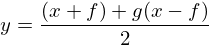<<compute_gain>>=
out = ((in + y) + eq->gain*(in - y)) * 0.5;

Where $y$ is the output, $x$ is the input signal, $g$ is the gain amount, and f is the filtered version of x.

Update filter state. The output of the 2-pole filter becomes v, or $v(n - 1)$, and the previous v becomes v, or $v(n - 2)$.

<<update_filter_state>>=
eq->v = eq->v;
eq->v = v;